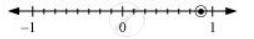# Draw the Number Line and Represent the Following Rational Numbers on It: 7/8 - Mathematics

Draw the number line and represent the following rational numbers on it:

7/8

#### Solution

7/8

This fraction represents 7 parts out of 8 equal parts. Therefore, each space between two integers on number line must be divided into 8 equal parts.

7/8 can be represent asConcept: Rational Numbers Between Two Rational Numbers
Is there an error in this question or solution?

#### APPEARS IN

NCERT Class 7 Maths
Chapter 9 Rational Numbers
Exercise 9.1 | Q 4.4 | Page 183